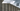# Valid Palindrome - Leet Code Solution

September 11, 2020## Problem Statement

Given a string, determine if it is a palindrome, considering only alphanumeric characters and ignoring cases.

Note: For the purpose of this problem, we define empty string as valid palindrome.

Example

``````Input: "A man, a plan, a canal: Panama"
Output: true

Input: "race a car"
Output: false``````

## Solution

1. Ignore case
2. Ignore any non-alphanumeric character

Lets run our simple two pointer system where:

• One pointer will start from left, while other start from extreme right end.
• Lets move left and right pointers untill they are pointing to a non-alphanumeric character
• Compare characters at both left and right position, check must be case-insensitive

### Code

``````public static boolean isAlphanumeric(char c) {
return Character.isDigit(c) || Character.isLetter(c);
}
public boolean isPalindrome(String s) {
if (s.length() == 0) return true;

int l = 0;
int r = s.length()-1;

while (l < r) {
while (!isAlphanumeric(s.charAt(l)) && l < r) {
l++;
}
while (!isAlphanumeric(s.charAt(r)) && l < r) {
r--;
}

if (Character.toLowerCase(s.charAt(l)) != Character.toLowerCase(s.charAt(r))) {
return false;
}

l++;
r--;
}

return true;
}``````

### Complexity

Its `O(n)`